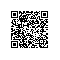# 把《c++ primer》读薄（3-2 标准库vector容器+迭代器初探）

#include <iostream>
#include <vector>
#include <string>
using std::string;
using std::vector;
using std::cout;
using std::cin;
using std::endl;

vector<int> ivec;//声明一个vector<int>数据类型的变量ivec

    vector<int> ivec1;//默认调无参构造
vector<int> ivec2(ivec1);//直接初始化ivec2为ivec1的一个副本
vector<string> strvec(ivec2);//error C2514: “std::vector”: 类没有构造函数。说明类型不一致，无法完成初始化！自然报错！

vector对象的初始化，vector类模版定义了四个构造函数，无参构造函数，直接初始化的带参构造函数，初始化为n个值为i的构造函数还有一种值初始化构造函数。

    vector<vector<int>> ivec;//ok，完全没问题！存储的是vector<int>类型的元素

    vector<int> ivec(20, 10);//初始化ivec为含有20个元素，每个元素=10
vector<string> svec(10, "hello");//初始化为含义10个元素，每个元是一个字符串hello

//值初始化方式
vector<int> ivec1(100);//内置类型，比如int类型的元素存在容器vector，那么默认初始化为100个0
vector<string> svec1(20);//同理若是类类型，如果有默认构造函数，那么按照它的默认构造函数初始化，比如这里是20个空串

    vector<string> svec(10, "null");
//vector::size_type len = svec.size();//error C2955: “std::vector”: 使用类 模板 需要 模板 参数列
cout << len << endl;

    vector<string>::size_type len = svec.size();
cout << len << endl;//打印10

    vector<int> ivec;
if (ivec.empty())
{
cout << ivec.size() << endl;//成功执行，打印0
}

    vector<string> svec;
string str;
//每次循环把输入的str字符串插入到vector容器对象的后面
while (cin >> str)
{
svec.push_back(str);
}
//直到循环结束为止

    vector<string> svec(10, "sss");
//重置容器内部元素的值为ooxx
for (vector<string>::size_type i = 0; i != svec.size(); i++)
{
svec[i] = "ooxx";
}

    vector<int> ivec;
for (vector<int>::size_type i = 0; i != 100; i++)
{
//ivec[i] = i;//这样做是错误的！程序中断！因为ivec是空的vector对象!下标操作只能针对已经存在的元素
ivec.push_back(i);//这样就对了，从尾部插入
}

    vector<int> ivec(10, 1);
//int i = ivec;//产生运行时错误，程序中断，不存在元素下标为10的

    int in;
vector<int> ivec;

begin:
cout << "请输入一组整数：注意ctrl+z结束输入！" << endl;

while (cin >> in)
{
ivec.push_back(in);
}
//判断空输入否
if (0 == ivec.size())
{
cout << "输入为空，重新输入！" << endl;
goto begin;
}

for (vector<int>::size_type i = 0; i < ivec.size(); i = i + 2)
{
cout << ivec[i] + ivec[i + 1] << "\t";
//每行输出的个数控制为5个。必须i+1，因为i初始值=0
if (0 == (i + 1) % 5)
{
cout << endl;
}
}

i<ivec.size()这里出错，下标溢出，只有元素个数是偶数的时候不错，奇数就溢出了。修改后：

 1 #include <iostream>
2 #include <vector>
3 #include <string>
4 using std::string;
5 using std::vector;
6 using std::cout;
7 using std::cin;
8 using std::endl;
9
10 int main(void)
11 {
12     int in;
13     vector<int> ivec;
14     cout << "请输入一组整数：注意ctrl+z结束输入！" << endl;
15
16     while (cin >> in)
17     {
18         ivec.push_back(in);
19     }
20     //判断空输入否
21     if (0 == ivec.size())
22     {
23         cout << "输入为空，重新输入！" << endl;
24         system("pause");
25         return -1;
26     }
27     //求相邻的元素的和，关键算法！
28     //因为是相邻元素，故判断完毕，i+2之后再赋给i，跨度为2
29     //且不知道输入的元素是奇数，肯丢留最后一个元素不计算，是偶数则正好计算完毕！
30     //这里必须是i < size -1，否则下标溢出错误，偶数没问题，主要是奇数的话，如果到倒数第二个元素还+2，必然是溢出错误！
31     for (vector<int>::size_type i = 0; i < ivec.size() - 1; i = i + 2)
32     {
33         cout << ivec[i] + ivec[i + 1] << "\t";
34         //每行输出的个数控制为5个。必须i+1，因为i初始值=0
35         if (0 == (i + 1) % 5)
36         {
37             cout << endl;
38         }
39     }
40     //判断奇偶
41     if (0 != ivec.size() % 2)
42     {
43         cout << "元素个数为奇数，最后一个元素" << ivec[ivec.size() - 1] << "被忽略！" << endl;
44     }
45
46     cout << endl;
47
48     system("pause");
49     return 0;
50 }1     vector<int> ivec;
2     int in;
3     cout << "输入一组正数，ctrl+z结束输入：" << endl;
4
5     while (cin >> in)
6     {
7         ivec.push_back(in);
8     }
9
10     if (0 == ivec.size())
11     {
12         cout << "空容器，必须输入元素！" << endl;
13         system("pause");
14         return -1;
15     }
16     //首末元素相加的处理，具有模版性质，也就是设计程序的通用性
17     //如果是奇数元素，那么中间的会留下，如果是偶数元素，没这个问题
18     vector<int>::size_type first = 0;
19     vector<int>::size_type last = 0;
20     vector<int>::size_type count = 0;//计数，控制打印输出
21
22     for (first = 0, last = ivec.size() - 1; first < last; first++, last--)
23     {
24         cout << ivec[first] + ivec[last] << "\t";
25         count++;
26         //控制打印输出每行3个数
27         if (0 == count % 3)
28         {
29             cout << endl;
30         }
31     }
32     //判断奇数还是偶数，给出提示
33     //灵活！使用简单的方式，如果是奇数的话，必然最后last和first重合
34     if (first == last)
35     {
36         cout << "中间的元素" << ivec[first] <<"留下了，因为元素个数是奇数！" << endl;
37     }
38
39     cout << endl;1 #include <iostream>
2 #include <vector>
3 #include <string>
4 #include <cctype>
5 using std::string;
6 using std::vector;
7 using std::cout;
8 using std::cin;
9 using std::endl;
10
11 int main(void)
12 {
13     vector<string> svec;
14     string str;
15
16     while (cin >> str)
17     {
18         svec.push_back(str);
19     }
20
21     if (0 == svec.size())
22     {
23         return -1;
24     }
25
26     for (vector<string>::size_type i = 0; i != svec.size(); i++)
27     {
28         for (string::size_type j = 0; j != svec[i].size(); j++)
29         {
30             //如果是小写字母
31             if (islower(svec[i][j]))
32             {
33                 //转换为大写输出
34                 svec[i][j] = toupper(svec[i][j]);
35             }
36         }
37
38         cout << svec[i] << "\t";
39
40         if (0 == (i + 1) % 5)
41         {
42             cout << endl;
43         }
44     }
45
46     system("pause");
47     return 0;
48 }//c++为每一种标准容器都定义了一种迭代器类型，迭代器是一种——可以检测容器内的元素并且可以遍历元素的数据类型。除了使用下标来访问vector对象之外，还可以使用迭代器访问，比下标操作更方便，更通用，因为所有的标准库的容器都支持迭代器，但是只有部分容器支持下标操作，故成熟的c++程序员应该使用迭代器，而不是下标操作访问容器内的元素。

//vector容器的迭代器类型
vector<int>::iterator iter;//定义一个iter变量，它的数据类型是vector<int>定义的迭代器类型

//记住，每个标准库容器都定义了自己的迭代器类型,用来遍历自己容器内的元素。

    //每个容器都定义了begin和end函数，目的是返回迭代器，如果容器不空，则begin返回的迭代器指向容器内的第一个元素
vector<int> ivec;
vector<int>::iterator iter;
iter = ivec.begin();//iter变量被初始化，使用容器的begin函数返回的迭代器，此时iter指该元素为ivec

//恰恰相反，end函数返回的迭代器，指向容器内末端元素的下一个元素！记住是下一个！不是最后一个！故end操作返回的也叫超出末端迭代器。说明end函数返回的迭代器指向一个不存在的元素，不指向容器内任何实际存在的元素！作用是哨兵！表示我们已经处理完毕容器所有元素！

    //新的赋值方式
vector<int> ivec(10, 2);
//把容器ivec的元素重置为0

//for (vector<int>::size_type i = 0; i != ivec.size(); i++)
//{
//    ivec[i] = 0;//这是old方法
//}

//比较经典常用的方法如下：
for (vector<int>::iterator iter = ivec.begin(); iter != ivec.end(); iter++)
{
*iter = 0;
}

//类似普通的const常量，但是有区别。比如，如果仅仅想遍历容器的元素，对迭代器有const_iterator类型的迭代器，对它解引用，得到的是指向const对象的引用

 1     string str;
2     vector<string> svec;
3
4     while (cin >> str)
5     {
6         svec.push_back(str);
7     }
8
9     for (vector<string>::const_iterator iter = svec.begin(); iter != svec.end(); iter++)
10     {
11         //*iter = "dada";//error，const_iterator类型的迭代器，本身可以被改变，比如自增，但是迭代器指向的容器内的元素不能被修改！
12         //和普通的const常量有一些区别！有些类似指向常量的指针，指针本身可以变，但是指向的内容不能修改。
13     }

1     vector<int> ivec(10);
2     //const类型的iter必须初始化
3     const vector<int>::iterator iter = ivec.begin();
4     //初始化之后不能被修改
5     //iter++;//error
6     //但是iter指向的内容可以被修改，对比，const_iterator类型的迭代器，类似指向常量的指针，迭代器本身能修改，指向的元素不能修改，，和他/她相反
7     *iter = 1;

const 迭代器是迭代器常量

const_iterator 是一种迭代器类型

 1     vector<int> ivec(10);
2     //const类型的iter必须初始化
3     vector<int>::iterator iter = ivec.begin();
4     //iter++;//ok
5     //iter--;//ok
6
7     //iter + 5;//ok,对一个迭代器对象加一个整型值，使得iter指向新的元素第6个元素，注意不要越界
8     //但是，加上或者减去的整型，最好是size_type类型的！
9
10     //iter - 100;//error,越界，程序中断！
11
12     //iter + 10;//ok,加的时候，可以加到最后一个元素的下一位。
13
14     //还能求两个迭代器的距离
15     vector<int>::iterator iter1 = ivec.end();
16
17     cout << iter1 - iter << endl;//打印10
18
19     //注意，这里相减得到的值，可能是负数，也可以是正数
20     cout << iter - iter1 << endl;//打印-10
21     //这就说明，这个值的类型不再是容器的size_type类型，而是新的容器的类型，叫：
22     //differenec_type
23     vector<int>::difference_type i = iter - iter1;//ok,是带符号类型

    iter1 + iter1;//报错！

vector<int>::iterator mid = (vi.begin() + vi.end())/2;

vector<int>::iterator mid = vi.begin() + vi.end()/2;

http://www.cnblogs.com/kubixuesheng/p/4138585.html使用钉钉扫一扫加入圈子
+ 订阅